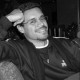You are currently browsing the tag archive for the ‘martin aigner’ tag.

Proofs from THE BOOK by Martin Aigner and Günter Ziegler begins by giving six proofs of the infinity of primes. We will go over the third proof. Before we go over this proof, lets cover some background.

— 1. Fermat Numbers —

Fermat numbers are defined by$\displaystyle F_{n}=2^{2^{n}}+1,$

so that${F_{0}=3,F_{1}=5,F_{2}=17,F_{3}=257,}$ and${F_{4}=65537}$. They are of great interest in many ways: for example, it was proved by Gauss that, if${F_{n}}$, is a prime${p}$, then a regular polygon of${p}$ sides can be inscribed in a circle by Euclidean methods. The property of the Fermat numbers which is relevant here is

Theorem 1 No two Fermat numbers have a common divisor greater than 1.

We will prove this theorem later.

The first four Fermat numbers are prime, and Fermat conjectured that all were prime. Euler, however, found in 1732 that$\displaystyle F_{5}=2^{2^{5}}+1=641\cdot6700417$

is composite.

In 1880 Landry proved that$\displaystyle F_{6}=2^{2^{6}}+1=274177\cdot67280421310721.$

It is currently known that${F_{n}}$, is composite for${5 \leq n \leq 32}$. Factoring Fermat numbers is extremely difficult as a result of their large size.${F_{12}}$ has${5}$ known factors with${C1187}$ remaining (where${C}$ denotes a composite number with$n$ digits).${F_{13}}$ has${4}$ known factors with${C2391}$ remaining.${F_{14}}$ has no known factors but is composite. There are currently four Fermat numbers that are known to be composite, but for which no single factor is known:${F_{14},F_{20},F_{22},}$ and${F_{14}}$. In all the other cases proved to be composite a factor is known. No prime${F_{n}}$ has been found beyond${F_{4}}$, and it seems unlikely that any more will be found using current computational methods and hardware.

— 2. Infinitude of Primes Theorem —

We are now ready to prove Euclid’s Second Theorem, also called the Infinitude of Primes Theorem using the third proof in Proofs from THE BOOK.

Theorem 2 (Euclid’s Second Theorem) The number of primes is infinite.

Proof: Next let us look at the Fermat numbers${F_{n}=2^{2^{n}}+1}$ for${n=0,1,2,\cdots}$. We will show that any two Fermat numbers are relatively prime (Theorem 1); hence there must be infinitely many primes. To this end, we verify the recursion$\displaystyle \prod_{k=0}^{n-1}F_{k}=F_{n}-2\quad(n\geq1),$

from which our assertion follows immediately. Indeed, if${m}$ is a divisor of, say,${F_{k}}$ and${F_{n}}$${(k, then${m}$ divides${2}$, and hence${m=1}$ or${2}$. But${m=2}$ is impossible since all Fermat numbers are odd.

To prove the recursion we use induction on${n}$. For${n=1}$ we have${F_{0}=3}$ and${F_{1}-2=5-2=3}$. With induction we now conclude$\displaystyle \begin{array}{rcl} \prod_{k=0}^{n} F_{k} & = & \left(\prod_{k=0}^{n-1}F_{k}\right)F_{n}\\ & = & (F_{n}-2)F_{n}\\ & = & (2^{2^{n}}-1)(2^{2^{n}}+1)\\ & = & 2^{2^{n+1}}-1\\ & = & F_{n+1}-2. \Box \end{array}$

I have re-posted this to test my changes to the latex2wp.py and terrystyle.py programs, compiled with Python Software Foundation’s Python 2.7.2 64 bit version, to add support for LyX 1.6.9. The code change incorporates some additional theorem-like environments, macros, font styles, and the numbering has been change so that the different theorem-like types each have a separate counter (e.g., theorem 1, theorem 2, lemma 1, proposition 1, theorem 3, lemma 2, …, as opposed to theorem 1, theorem 2, lemma 3, proposition 4, …). Furthermore, I have provided more background information which will benefit those without a background in abstract algebra.

Proofs from THE BOOK by Martin Aigner and Günter Ziegler begins by giving six proofs of the infinity of primes. The first proof is what they call "the oldest Book Proof" attributed to Euclid. Before we go over this proof, lets cover some background. Read the rest of this entry »

Proofs from THE BOOK by Martin Aigner and Günter Ziegler begins by giving six proofs of the infinity of primes. The first proof is what they call “the oldest Book Proof” attributed to Euclid. Before we go over this proof, lets cover some background. Read the rest of this entry »

“You don’t have to believe in God, but you have
to believe in The Book.”—Paul Erdős

Paul Erdős liked to talk about The Book, in which God maintains the perfect proofs for mathematical theorems, following the dictum of G. H. Hardy that there is no permanent place for ugly mathematics.

JOEL SPENCER: “Paul talks about The Book. The Book has all the theorems of mathematics. Theorems can be proven in a lot of different ways, but in The Book there is only one proof and it is the one that is the clearest proof, the one that gives the most insight, the most aesthetic proof. It’s what he calls The Book proof. And sometimes when there’s a problem and somebody solves it and the proof is not so beautiful, then he’ll say, “Well okay, but let’s look for The Book proof; let’s try to find The Book proof.” And this is the sense of mathematics, that…that The Book is there, the theorems have an existence of their own. And what we’re doing is we’re just trying to uncover. We’re trying to read the pages of The Book. We don’t create mathematics. What we do is we read the pages of The Book. We discover the pages of The Book. So when he goes from university to university, and he talks about problems, and he asks everybody to try to solve these problems, it doesn’t matter who solves the problem. It really doesn’t matter to him, because all of us are in the same venture. We’re all just trying to uncover the pages. And sometimes we succeed. Sometimes we find these beautiful theorems.”
View the video of Joel Spencer describing The Book here.

Proofs from THE BOOK is an effort by Martin Aigner and Günter Ziegler to reveal an approximation to a portion of The Book